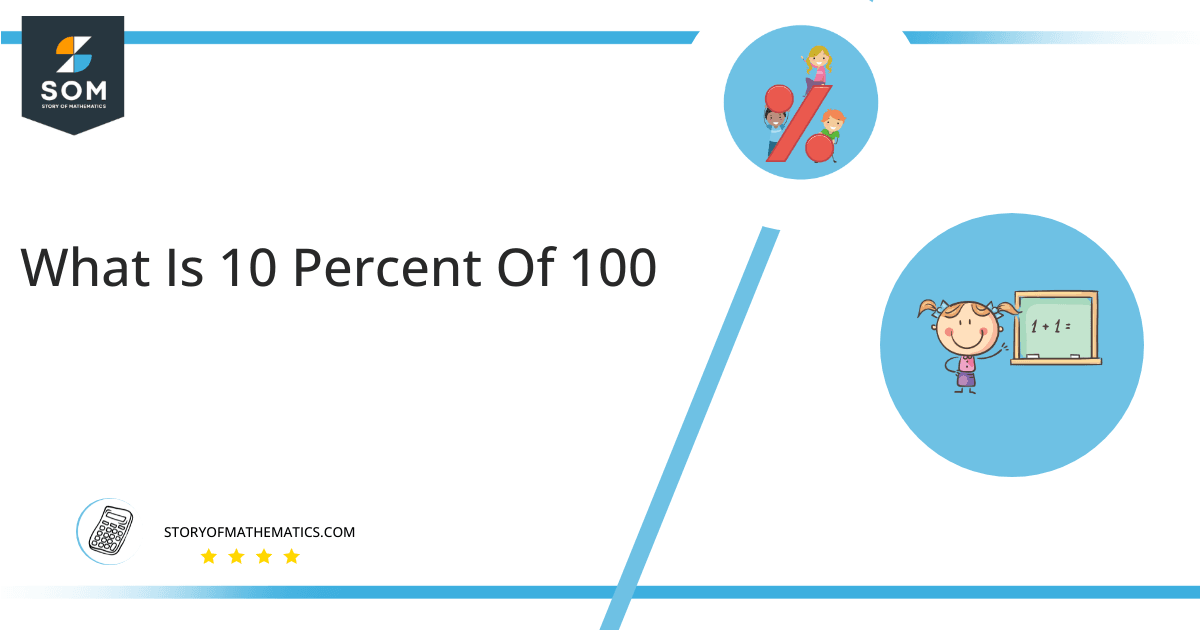# What is 10 percent of 100 + Solution with Free Steps10% of 100 is equivalent to 10. This solution may be calculated by multiplying the fraction 0.10 and number 100.

The calculation of 10% of 100 is useful in many applications. Let us say that you school is arranging a field trip to a hill station but due to scarcity of funds only the top 10% of students will be taken from each class comprising of 100 students. Now since you know that 10% of 100 equals 10, you will quickly jump to the conclusion that only 10 students will go on the field trip out of 100.

In this article, our aim is to find the 10 percent of 100 which is a percentage value.

## What Is 10 percent of 100?

10 percent of 100 equals 10. This solution can be obtained by multiplying the factor 0.10 with 100.

The solution to the problem of 10% of 100 can be obtained through the multiplication of the fractional value 1/10 with the number 100 which is then further simplified to obtain the final answer that is 10. We can calculate the percentage values by following such simple arithmetic steps.

## How To Calculate 10% of 100?

We can calculate the 10% value of 100 by following some simple arithmetic steps given below:### Step 1

Firstly, depicting 10 percent of 100 in the form of a mathematical equation as:

= 10% x 100

### Step 2

Replacing the 1/100 fractional value with % sign, above expression changes:

= 10 x ( 1 / 100 ) x 100

### Step 3

Using the arithmetic simplification:

= ( 10 x 100 ) / 100

Multiplying 10 and 100:

= 1000 / 100

Dividing 1000 by 100:

= 10

The following pie chart shows 10 percent of 100.Figure 1: Pie Chart of 10 percent of 100

The above figure shows two parts. The orange slice of the pie chart shows the 10% value of a hundred, which is equal to the value of 10 on an absolute scale. The green slice of the pie chart shows the 90% remaining value of a hundred, which is equal to 90 on an absolute scale. The total area of the pie chart is equal to 100.

The method in which we have to find a depiction of a given unique number in such a way that it could be divided into 100 fractions is known as the percentage method. A percentage is nothing but a ratio of two numerals. It’s a dimensionless quantity.

Therefore, 10 percent of 100 is equivalent to 10.

All the Mathematical drawings/images are created using GeoGebra.